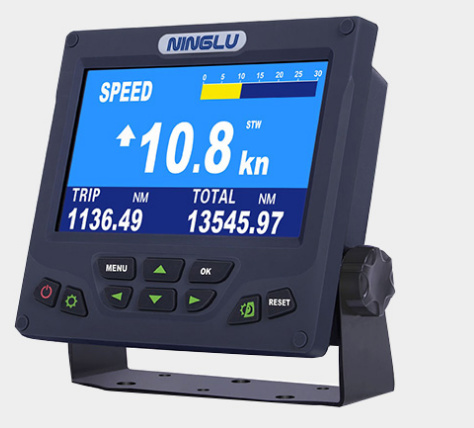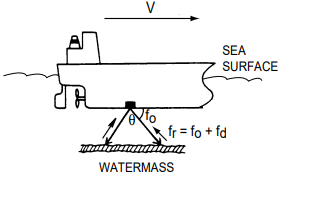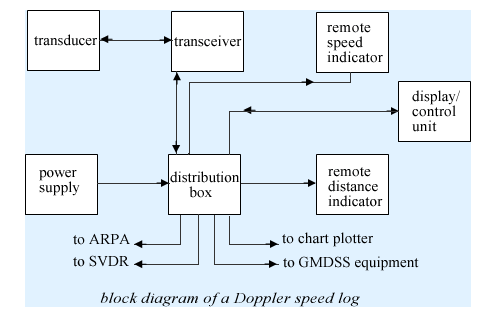# How does Doppler speed log measures ship’s speed?

The Doppler speed log measures ship’s speed by using the Doppler Effect, which is observed as a frequency shift resulting from relative motion between a transmitter and receiver or reflector of acoustic or electromagnetic energy.All ships of 300 GRT and upwards and all passenger ships irrespective of size shall be with a with a speed and distance measuring device, means, means , to indicate speed and distance through water.

## What is the working principle of the doppler speed log?

The Doppler speed log measures ship’s speed by using the Doppler Effect, which is observed as a frequency shift resulting from relative motion between a transmitter and receiver or reflector of acoustic or electromagnetic energy.

Doppler log uses the principle of Doppler shift to calculate the speed through the water. A wave transmitter is installed at the bottom of the ship which transmits waves at an angle (usually 60 degrees) to the ship’s keel.The Doppler Speed log has a pair-beam, one directed in the fore direction and the other in the aft direction, which emits ultrasonic waves at an angle of θ to the waterline towards ship’s fore and aft directions. The frequency of the received signal is then compared with that of the transmitted frequency to measure the Doppler shift to calculate the ship’s speed.

The relative motion causes the Doppler shift and the ultrasonic waves reflected at the watermass (plankton or any underwater objects) are received at the frequency of fr = fo + fd where fd is the number of frequency shifts counted at the receiver circuit.

### To calculate the ship’s speed, the following formula is used.

V = fd/fo • c/2sinθ

c: Underwater velocity

fd: doppler shift frequency

fo: original transmitted frequency

V: relative speed in the direction of transmitted sound wave

Note that the sound velocity in water changes with water temperature and water pressure but the Doppler speed readout is automatically compensated for change by using a temperature sensor.

As we would know the angle of the beam with respect to the keel, a co-relation can be drawn between this speed and the actual speed of the ship in the forward direction.

Transceiver will have both the frequencies f(d) and f(o) known. We know the sound wave velocity in water.

The only unknown is the speed of the vessel (V) which Doppler log can calculate easily with the above formula.

## Block diagram of doppler speed log